# Sequence pattern 2 - Consistent difference between differences

The next easiest pattern in a sequence to find is to look for a consistent difference between differences.

They also call this a quadratic sequence. (For a definition of quadratics see our mammoth memory quadratics section)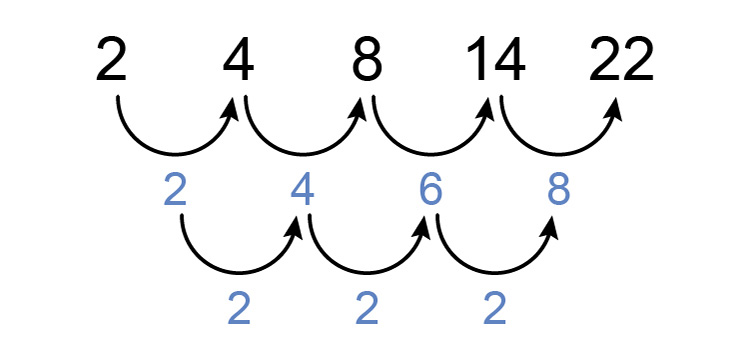There is a consistent difference between the differences of the original sequence and this is 2.

Quadratic =x^2  Involved here (something to the power of 2)

Example 1

Find the pattern in the following sequence:

7,  9,  13,  19,  27

First, see if there is a consistent difference between each number.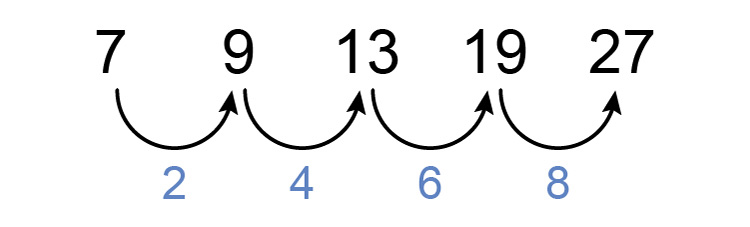There is not but now see if there is a constant difference between differences.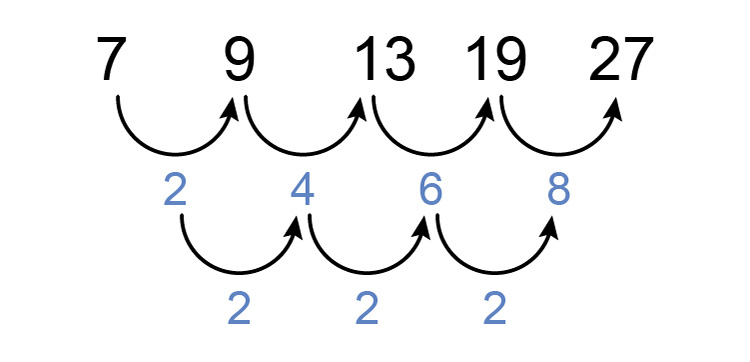We can see that there is.

Answer = 2

Example 2

Find the pattern in the following sequence:

5,  12,  25,  44,  69

First, see if there is a consistent difference between each number.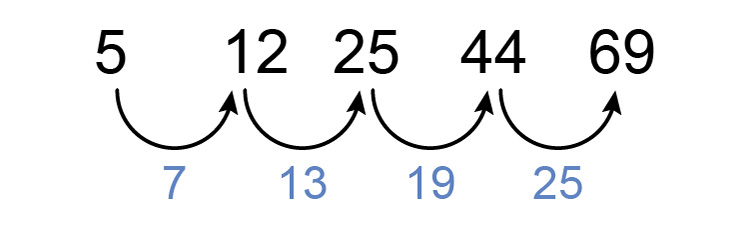There is not but now see if there is a constant difference between differences.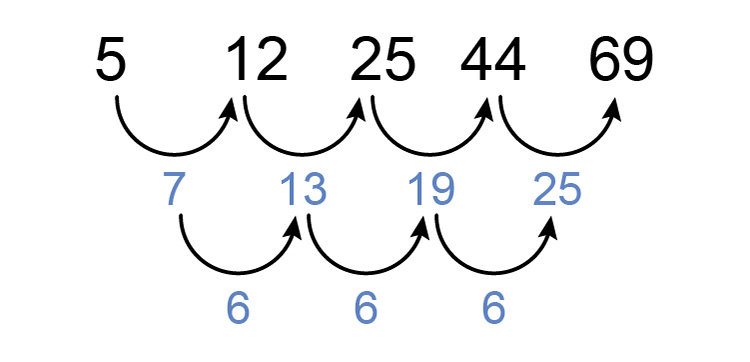We can see that there is.

Answer = 6

Example 3

Find the pattern in the following sequence:

-3,  3,  13,  27,  45

First, see if there is a consistent difference between each number.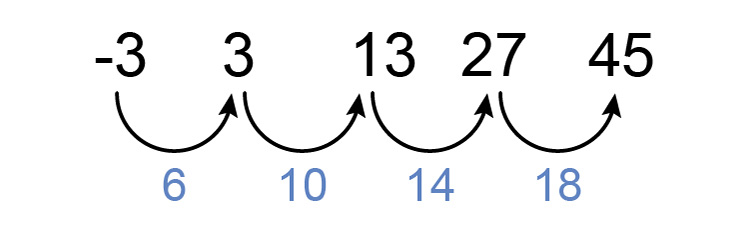There is not but now see if there is a constant difference between differences.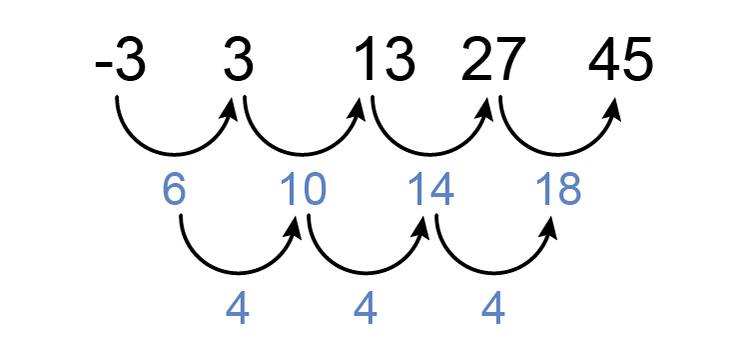We can see that there is.

Answer = 4

Example 4

Find the pattern in the following sequence:

0,  0,  2,  6,  12

First, see if there is a consistent difference between each number.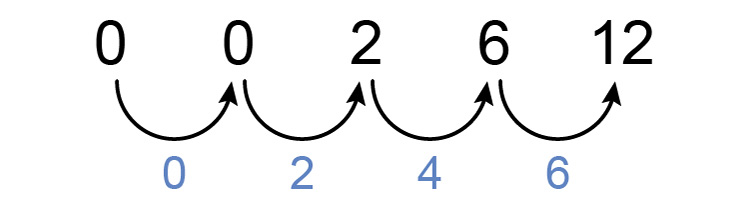There is not but now see if there is a constant difference between differences.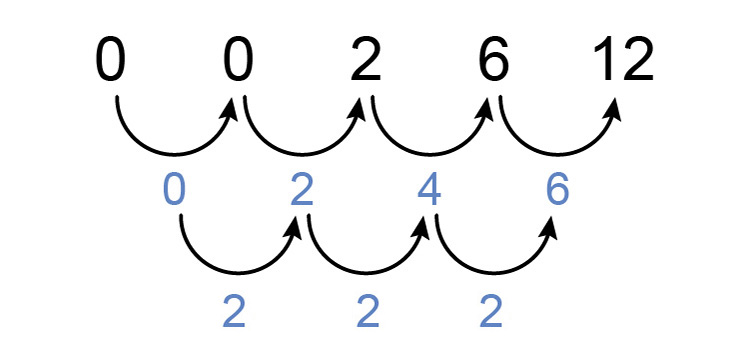We can see that there is.

Answer = 2

Example 5

Find the pattern in the following sequence:

1,  11,  20,  28,  35

First, see if there is a consistent difference between each number.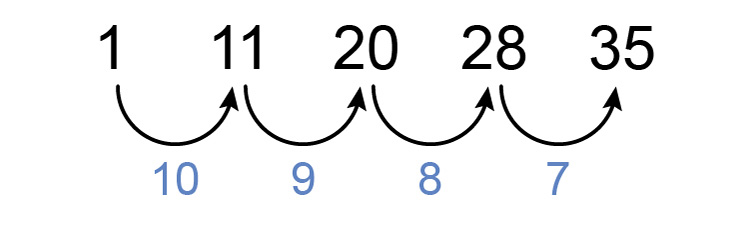There is not but now see if there is a constant difference between differences.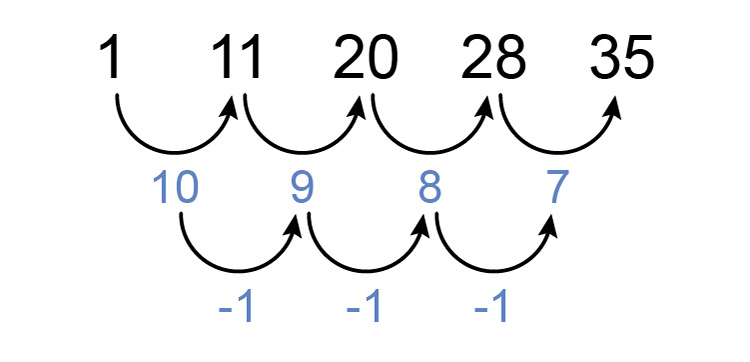We can see that there is.

Answer = -1